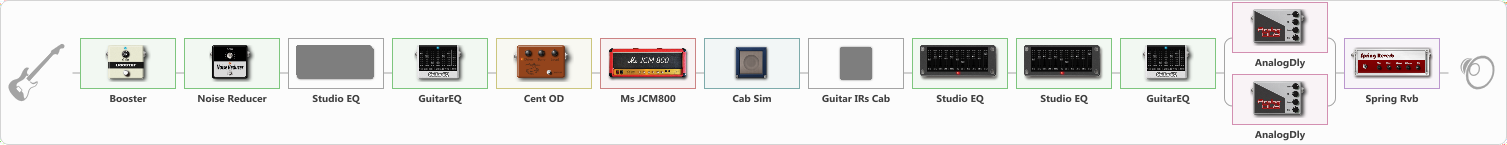Discussion in 'ToneLib-GFX presets' started by levitation, Apr 20, 2020.

Preset name: Erik Lead 2 (I'am Alright neilzaza)

this is my second tone before 30 day trial, now 28 day in trial, i use the backing track neil zaza i'm alright and steve vai the Love of God, i don't know guys, the tone for myself its enough.
but if you want to get steve sound, u must add another eq to get hight frequency, i mean, the eq not from this fx

Effects chain:Effect: "Booster" (Dynamics / Filter), active - "yes"
{

"Gain" = 61
}

Effect: "Noise Reducer" (Dynamics / Filter), active - "yes"
{

"Sens" = 55
"Mode" = Soft
}

Effect: "Studio EQ" (Dynamics / Filter), active - "no"
{

"31 Hz" = 0
"62 Hz" = 0
"125 Hz" = 0
"250 Hz" = 0
"500 Hz" = 0
"1 kHz" = 4
"2 kHz" = 3
"4 kHz" = 6
"8 kHz" = -3
"16 kHz" = 0
"Level (dB)" = 0
}

Effect: "GuitarEQ" (Dynamics / Filter), active - "yes"
{

"160 Hz" = 0
"400 Hz" = 0
"800 Hz" = 0
"1.6 kHz" = 0
"3.2 kHz" = 0
"6.4 kHz" = 9
"12 kHz" = 0
"Level (dB)" = 5
}

Effect: "Cent OD" (Overdrive / Distortion), active - "yes"
{

"Drive" = 10
"Tone" = 89
"Level" = 84
}

Effect: "Ms JCM800" (Amp simulators), active - "yes"
{

"Gain" = 64
"Bass" = 55
"Middle" = 46
"Treble" = 69
"Presence" = 30
"Master" = 52
"Level (dB)" = 9
}

Effect: "Cab Sim" (Cabinets), active - "yes"
{

"Model" = 4x12" Greenback
"Level (dB)" = 0
}

Effect: "Guitar IRs Cab" (Cabinets), active - "no"
{

"Model" = Mesa Rectifier (4x12")
"Mic Position" = Middle
"Mic Distance" = Middle
"Low Cut (Hz)" = 78
"Hi Cut (kHz)" = 20.0
"Mix" = 25
"Level (dB)" = -2
}

Effect: "Studio EQ" (Dynamics / Filter), active - "yes"
{

"31 Hz" = 0
"62 Hz" = 0
"125 Hz" = 0
"250 Hz" = 0
"500 Hz" = 1
"1 kHz" = -2
"2 kHz" = 0
"4 kHz" = -2
"8 kHz" = 2
"16 kHz" = 0
"Level (dB)" = 2
}

Effect: "Studio EQ" (Dynamics / Filter), active - "yes"
{

"31 Hz" = -3
"62 Hz" = -3
"125 Hz" = 0
"250 Hz" = 0
"500 Hz" = 0
"1 kHz" = 0
"2 kHz" = 0
"4 kHz" = -4
"8 kHz" = -4
"16 kHz" = -13
"Level (dB)" = -1
}

Effect: "GuitarEQ" (Dynamics / Filter), active - "yes"
{

"160 Hz" = -2
"400 Hz" = -2
"800 Hz" = 2
"1.6 kHz" = 0
"3.2 kHz" = -5
"6.4 kHz" = -6
"12 kHz" = -8
"Level (dB)" = 1
}

Effect: "Splitter" (Dynamics / Filter), active - "yes"
{

"A-Bypass" = Off
"A-Pan" = -49
"A-Level" = 55
"B-Bypass" = Off
"B-Pan" = 46
"B-Level" = 55
'A' branch:
{

Effect: "AnalogDly" (Delay), active - "yes"
{

"Time" = 452
"Feedback" = 52
"Tone" = 61
"Mix" = 57
}
}
'B' branch:
{

Effect: "AnalogDly" (Delay), active - "yes"
{

"Time" = 812
"Feedback" = 59
"Tone" = 56
"Mix" = 57
}
}
}

Effect: "Spring Rvb" (Reverb), active - "yes"
{

"Time" = 7.0
"PreDelay" = 0
"LoDamp" = 16
"HiDamp" = 26
"Mix" = 47
}

Note: You will need to download and install the ToneLib-GFX software to use the preset.